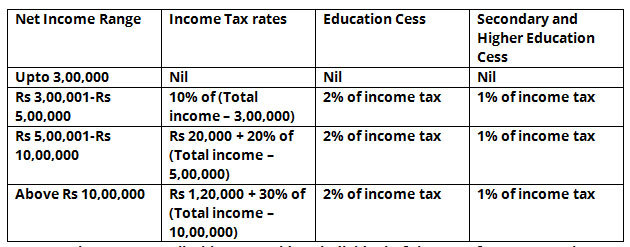# Java Program to Calculate Income Tax | Java Programs

Java code to calculate income tax for company or for employee –  The following income tax calculator on java has been written in 4 different ways. Suitable examples and sample programs have been included in order to make you understand simply.

• Using Scanner Class
• Using a Static Method
• Using Separate Class
• Using Command-Line Arguments

Income varies from person to person depending on their ranges of yearly income.As you can see, these are the most basic slabs in the application as far as the Indian Government is concerned. Those are as follows:

• Up to 3-LPA
• Rs. 300001 – Rs. 500000
• Rs. 500001 – Rs. 1000000
• Rs. 1000000 and above

## Java Income Tax – Using Scanner Class

To find the income tax, we will first require to know the income as, based on the range in which the income falls, the amount to be paid and the calculations vary.

To get the input of income at runtime, we make use of the Scanner class of Java. With this, the user can give inputs in the console screen itself and doesn’t require to come to the code part.

After getting the value of income, we’ll have to figure out in which range it falls. Based on this condition, the formula to be used to determine the income tax varies as stated in the above table.

So, based on our input income, if checks which of the above conditions satisfy and following that formula we derive our resultant output income tax.

Output:

Using Static Method

In the above Java method, the entire logic along with input output statements were written within the main method itself.

Let us consider that, in future we would like to make use of the same logic somewhere else in the code. Under that situation the same set of statements have to be rewritten again.

To avoid this, if the set of statements containing the main logic of the code (discussed in the above method) is placed in a separate block or what is called the static method (incomeTax) then, just making a function call of this method would be sufficient and by passing the arguments for which the calculation is to be made.

Thereby, making use of a static method helps us reuse a block of statements wherever required.

Output:

### Using Command Line Arguments

In all the above methods used to solve this problem, we have made use of the Scanner class to read input at runtime. In this method we’ll see another way to read input at runtime. This method is nothing but, making use of command line arguments

Here, while we give the run command, after the name of the Java code to be run we’ll pass input arguments (arg) with a space in between. As we require only one input i.e., income, we’ll pass only single argument.

This argument is of string type so, we’ll convert it into our desired type i.e., double by parsing it as follows:

This value is stored in a variable and acts as our input. Now that the input value is read, we’ll follow the same set of instructions as given above to calculate the resultant output income tax.

Output:

x

## Plus Star Pattern Java Program | Patterns

Java program to print plus star pattern program – We have written the below print/draw ...